# Example: Charts with Data Tools#

A demo of an various Excel chart data tools that are available via an XlsxWriter chart. These include, Trendlines, Data Labels, Error Bars, Drop Lines, High-Low Lines and Up-Down Bars.

Chart 1 in the following example is a chart with trendlines: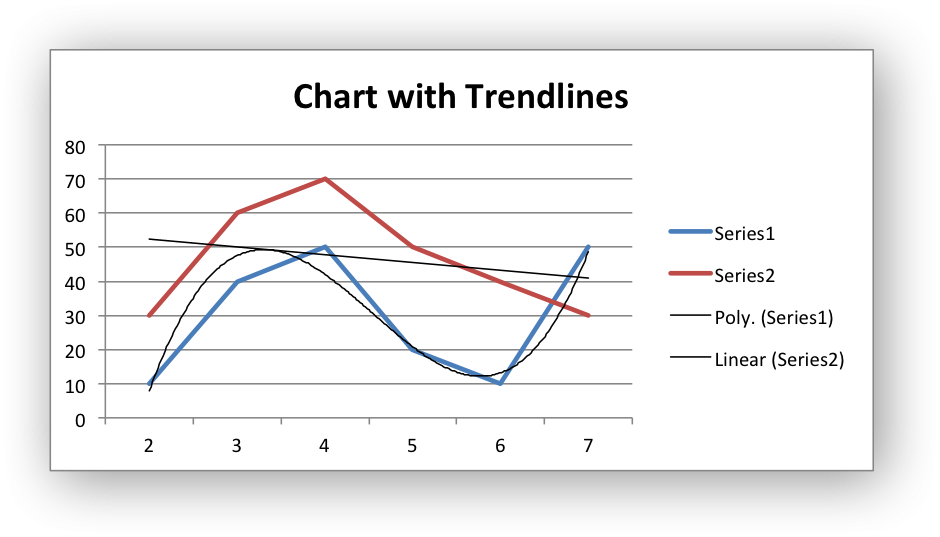Chart 2 is a chart with data labels and markers: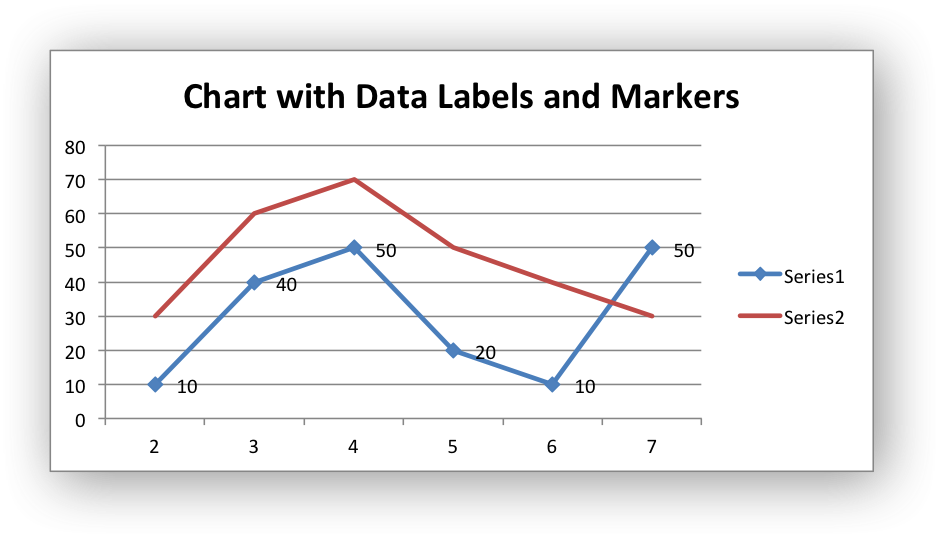Chart 3 is a chart with error bars: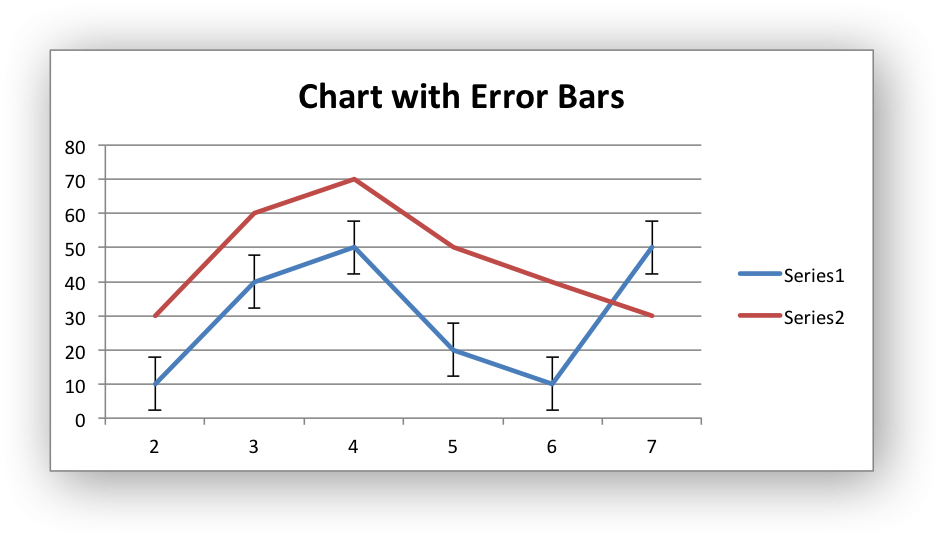Chart 4 is a chart with up-down bars: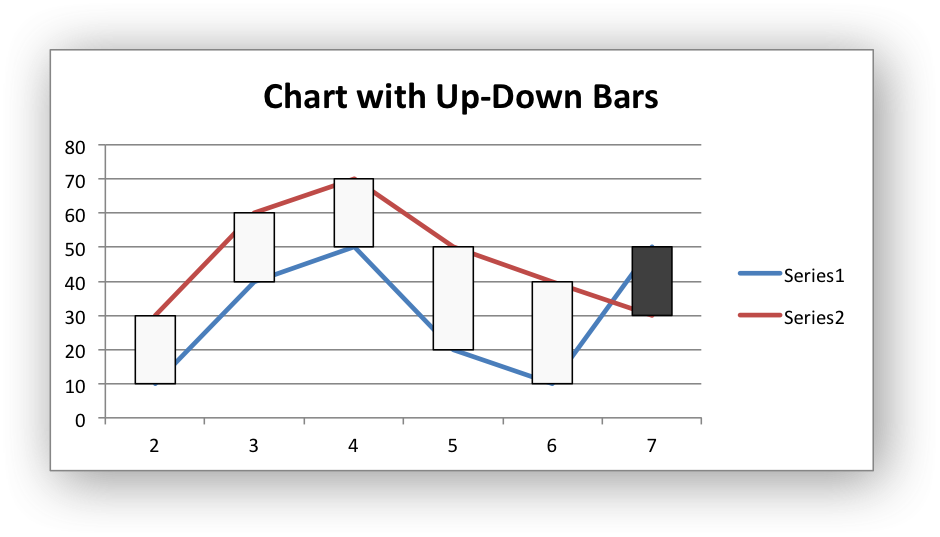Chart 5 is a chart with hi-low lines: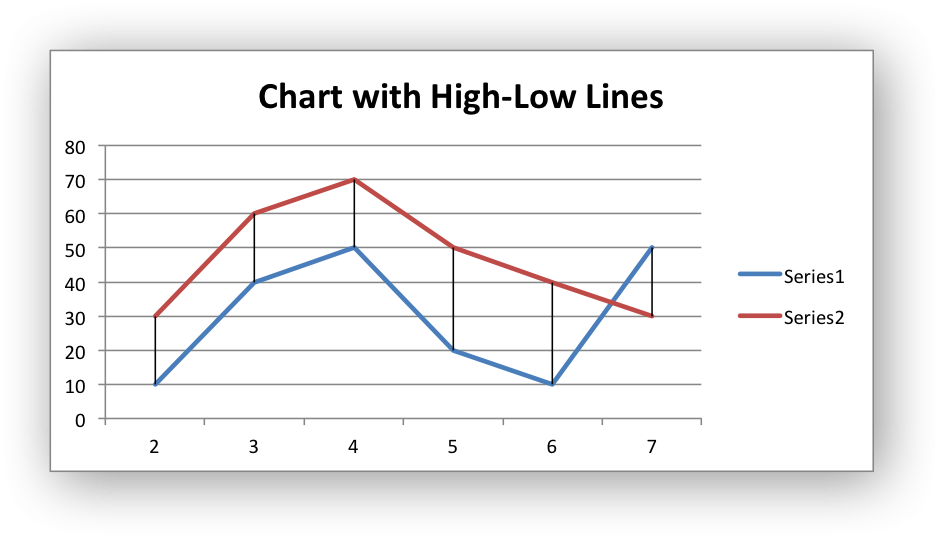Chart 6 is a chart with drop lines: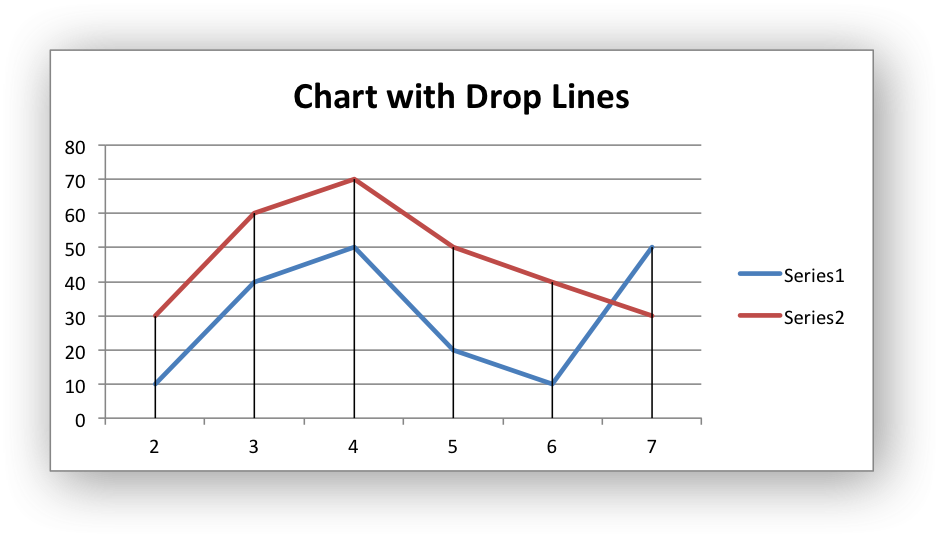```#######################################################################
#
# A demo of an various Excel chart data tools that are available via
# an XlsxWriter chart.
#
# These include, Trendlines, Data Labels, Error Bars, Drop Lines,
# High-Low Lines and Up-Down Bars.
#
# Copyright 2013-2023, John McNamara, jmcnamara@cpan.org
#
import xlsxwriter

workbook = xlsxwriter.Workbook("chart_data_tools.xlsx")

# Add the worksheet data that the charts will refer to.
headings = ["Number", "Data 1", "Data 2"]
data = [
[2, 3, 4, 5, 6, 7],
[10, 40, 50, 20, 10, 50],
[30, 60, 70, 50, 40, 30],
]

worksheet.write_column("A2", data)
worksheet.write_column("B2", data)
worksheet.write_column("C2", data)

#######################################################################
#
# Trendline example.
#
# Create a Line chart.

# Configure the first series with a polynomial trendline.
{
"categories": "=Sheet1!\$A\$2:\$A\$7",
"values": "=Sheet1!\$B\$2:\$B\$7",
"trendline": {
"type": "polynomial",
"order": 3,
},
}
)

# Configure the second series with a moving average trendline.
{
"categories": "=Sheet1!\$A\$2:\$A\$7",
"values": "=Sheet1!\$C\$2:\$C\$7",
"trendline": {"type": "linear"},
}
)

chart1.set_title({"name": "Chart with Trendlines"})

# Insert the chart into the worksheet (with an offset).
worksheet.insert_chart("D2", chart1, {"x_offset": 25, "y_offset": 10})

#######################################################################
#
# Data Labels and Markers example.
#
# Create a Line chart.

# Configure the first series.
{
"categories": "=Sheet1!\$A\$2:\$A\$7",
"values": "=Sheet1!\$B\$2:\$B\$7",
"data_labels": {"value": 1},
"marker": {"type": "automatic"},
}
)

# Configure the second series.
{
"categories": "=Sheet1!\$A\$2:\$A\$7",
"values": "=Sheet1!\$C\$2:\$C\$7",
}
)

chart2.set_title({"name": "Chart with Data Labels and Markers"})

# Insert the chart into the worksheet (with an offset).
worksheet.insert_chart("D18", chart2, {"x_offset": 25, "y_offset": 10})

#######################################################################
#
# Error Bars example.
#
# Create a Line chart.

# Configure the first series.
{
"categories": "=Sheet1!\$A\$2:\$A\$7",
"values": "=Sheet1!\$B\$2:\$B\$7",
"y_error_bars": {"type": "standard_error"},
}
)

# Configure the second series.
{
"categories": "=Sheet1!\$A\$2:\$A\$7",
"values": "=Sheet1!\$C\$2:\$C\$7",
}
)

chart3.set_title({"name": "Chart with Error Bars"})

# Insert the chart into the worksheet (with an offset).
worksheet.insert_chart("D34", chart3, {"x_offset": 25, "y_offset": 10})

#######################################################################
#
# Up-Down Bars example.
#
# Create a Line chart.

chart4.set_up_down_bars()

# Configure the first series.
{
"categories": "=Sheet1!\$A\$2:\$A\$7",
"values": "=Sheet1!\$B\$2:\$B\$7",
}
)

# Configure the second series.
{
"categories": "=Sheet1!\$A\$2:\$A\$7",
"values": "=Sheet1!\$C\$2:\$C\$7",
}
)

chart4.set_title({"name": "Chart with Up-Down Bars"})

# Insert the chart into the worksheet (with an offset).
worksheet.insert_chart("D50", chart4, {"x_offset": 25, "y_offset": 10})

#######################################################################
#
# High-Low Lines example.
#
# Create a Line chart.

chart5.set_high_low_lines()

# Configure the first series.
{
"categories": "=Sheet1!\$A\$2:\$A\$7",
"values": "=Sheet1!\$B\$2:\$B\$7",
}
)

# Configure the second series.
{
"categories": "=Sheet1!\$A\$2:\$A\$7",
"values": "=Sheet1!\$C\$2:\$C\$7",
}
)

chart5.set_title({"name": "Chart with High-Low Lines"})

# Insert the chart into the worksheet (with an offset).
worksheet.insert_chart("D66", chart5, {"x_offset": 25, "y_offset": 10})

#######################################################################
#
# Drop Lines example.
#
# Create a Line chart.

chart6.set_drop_lines()

# Configure the first series.
{
"categories": "=Sheet1!\$A\$2:\$A\$7",
"values": "=Sheet1!\$B\$2:\$B\$7",
}
)

# Configure the second series.
{
"categories": "=Sheet1!\$A\$2:\$A\$7",
"values": "=Sheet1!\$C\$2:\$C\$7",
}
)